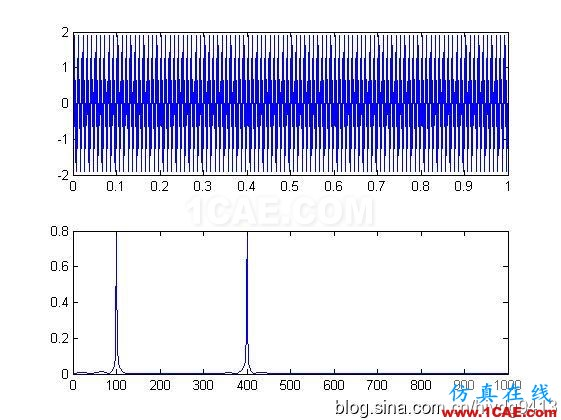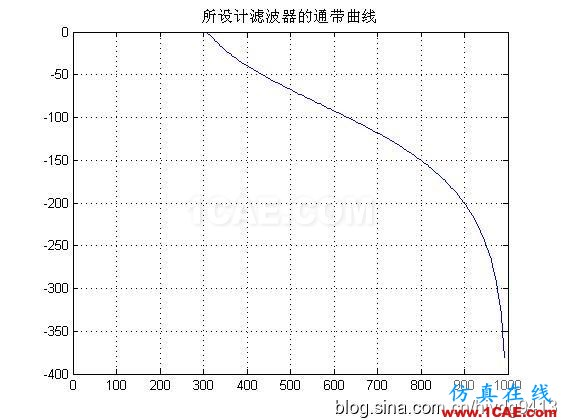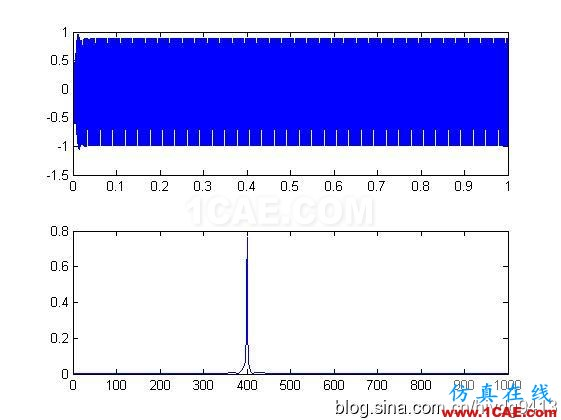# [转载]分享matlab程序之——滤波器篇（高通，低通）

2017-01-15  by:CAE仿真在线  来源:互联网

1.低通滤波器

function y=lowp(x,f1,f3,rp,rs,Fs)
%低通滤波
%使用注意事项:通带或阻带的截止频率的选取范围是不能超过采样率的一半
%即,f1,f3的值都要小于 Fs/2
%x:需要带通滤波的序列
% f 1:通带截止频率
% f 3:阻带截止频率
%rp:边带区衰减DB数设置
%rs:截止区衰减DB数设置
%FS:序列x的采样频率
% rp=0.1;rs=30;%通带边衰减DB值和阻带边衰减DB值
% Fs=2000;%采样率
%
wp=2*pi*f1/Fs;
ws=2*pi*f3/Fs;
% 设计切比雪夫滤波器;
[n,wn]=cheb1ord(wp/pi,ws/pi,rp,rs);
[bz1,az1]=cheby1(n,rp,wp/pi);
%查看设计滤波器的曲线
[h,w]=freqz(bz1,az1,256,Fs);
h=20*log10(abs(h));
figure;plot(w,h);title('所设计滤波器的通带曲线');grid on;
%
y=filter(bz1,az1,x);%对序列x滤波后得到的序列y
end

--------------------------------------

fs=2000;
t=(1:fs)/fs;
ff1=100;
ff2=400;
x=sin(2*pi*ff1*t)+sin(2*pi*ff2*t);
figure;
subplot(211);plot(t,x);
subplot(212);hua_fft(x,fs,1);
%低通测试
% y=filter(bz1,az1,x);
y=lowp(x,300,350,0.1,20,fs);
figure;
subplot(211);plot(t,y);
subplot(212);hua_fft(y,fs,1);%hua_fft()函数是画频谱图的函数,代码在下面给出,要保存为m文件调用

%这段例子还调用了我自己写的专门画频谱图的函数,也给出,不然得不出我的结果

%画信号的幅频谱和功率谱
%频谱使用matlab例子表示
function hua_fft(y,fs,style,varargin)
%当style=1,画幅值谱;当style=2,画功率谱;当style=其他的,那么花幅值谱和功率谱
%当style=1时,还可以多输入2个可选参数
%可选输入参数是用来控制需要查看的频率段的
%第一个是需要查看的频率段起点
%第二个是需要查看的频率段的终点
%其他style不具备可选输入参数,如果输入发生位置错误
nfft= 2^nextpow2(length(y));%找出大于y的个数的最大的2的指数值(自动进算最佳FFT步长nfft)
%nfft=1024;%人为设置FFT的步长nfft
y=y-mean(y);%去除直流分量
y_ft=fft(y,nfft);%对y信号进行DFT,得到频率的幅值分布
y_p=y_ft.*conj(y_ft)/nfft;%conj()函数是求y函数的共轭复数,实数的共轭复数是他本身。
y_f=fs*(0:nfft/2-1)/nfft;�T变换后对应的频率的序列
% y_p=y_ft.*conj(y_ft)/nfft;%conj()函数是求y函数的共轭复数,实数的共轭复数是他本身。
if style==1
if nargin==3
plot(y_f,2*abs(y_ft(1:nfft/2))/length(y));%matlab的帮助里画FFT的方法
%ylabel('幅值');xlabel('频率');title('信号幅值谱');
%plot(y_f,abs(y_ft(1:nfft/2)));%论坛上画FFT的方法
else
f1=varargin{1};
fn=varargin{2};
ni=round(f1 * nfft/fs+1);
na=round(fn * nfft/fs+1);
plot(y_f(ni:na),abs(y_ft(ni:na)*2/nfft));
end

elseif style==2
plot(y_f,y_p(1:nfft/2));
%ylabel('功率谱密度');xlabel('频率');title('信号功率谱');
else
subplot(211);plot(y_f,2*abs(y_ft(1:nfft/2))/length(y));
ylabel('幅值');xlabel('频率');title('信号幅值谱');
subplot(212);plot(y_f,y_p(1:nfft/2));
ylabel('功率谱密度');xlabel('频率');title('信号功率谱');
end
end2.高通滤波器

function y=highp(x,f1,f3,rp,rs,Fs)
%高通滤波
%使用注意事项:通带或阻带的截止频率的选取范围是不能超过采样率的一半
%即,f1,f3的值都要小于 Fs/2
%x:需要带通滤波的序列
% f 1:通带截止频率
% f 2:阻带截止频率
%rp:边带区衰减DB数设置
%rs:截止区衰减DB数设置
%FS:序列x的采样频率
% rp=0.1;rs=30;%通带边衰减DB值和阻带边衰减DB值
% Fs=2000;%采样率
%
wp=2*pi*f1/Fs;
ws=2*pi*f3/Fs;
% 设计切比雪夫滤波器;
[n,wn]=cheb1ord(wp/pi,ws/pi,rp,rs);
[bz1,az1]=cheby1(n,rp,wp/pi,'high');

%查看设计滤波器的曲线
[h,w]=freqz(bz1,az1,256,Fs);
h=20*log10(abs(h));
figure;plot(w,h);title('所设计滤波器的通带曲线');grid on;
y=filter(bz1,az1,x);
end

fs=2000;
t=(1:fs)/fs;
ff1=100;
ff2=400;
x=sin(2*pi*ff1*t)+sin(2*pi*ff2*t);
figure;
subplot(211);plot(t,x);
subplot(212);hua_fft(x,fs,1);

%------高通测试
z=highp(x,350,300,0.1,20,fs);
figure;
subplot(211);plot(t,z);
subplot(212);hua_fft(z,fs,1);• 客服在线请直接联系我们的客服，您也可以通过下面的方式进行在线报名，我们会及时给您回复电话，谢谢！